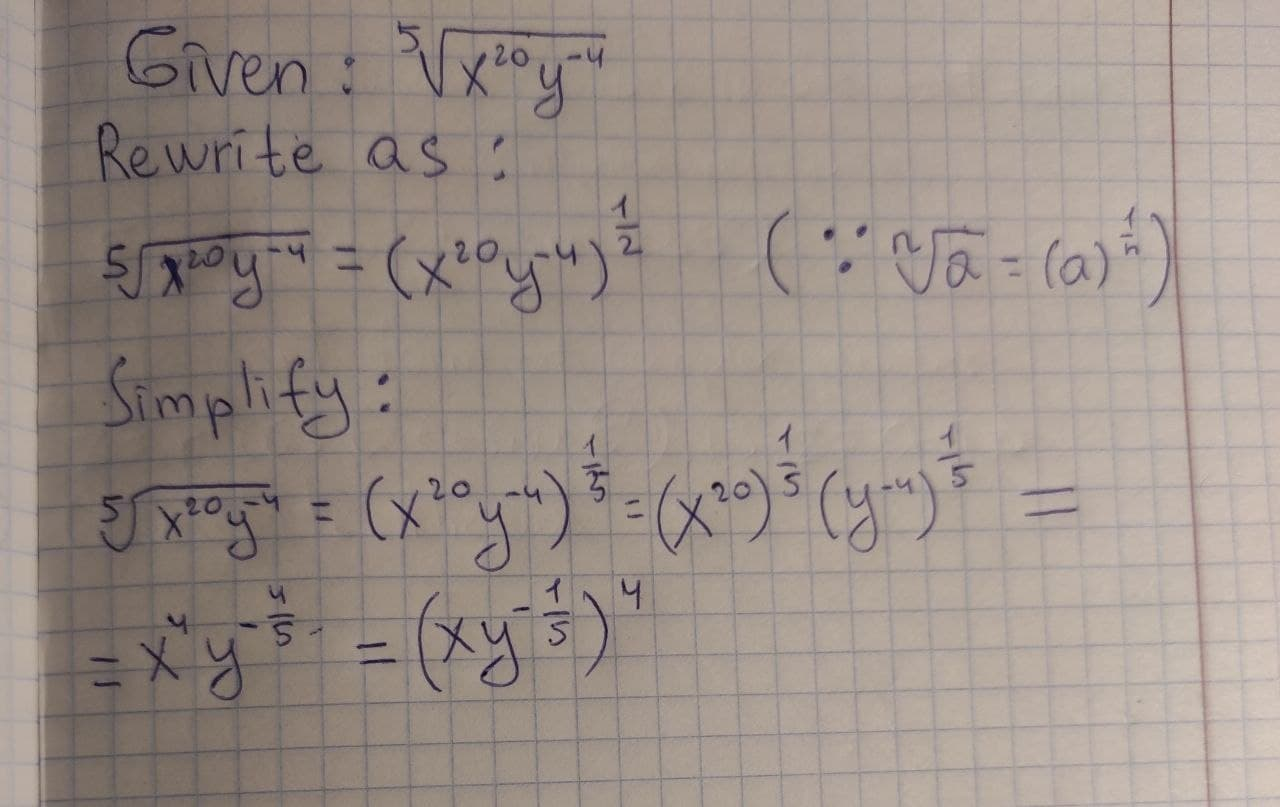# Rewrite the following expressions without using radicals or negative exponents. Simplify when possible. root(5)(x^20y^-4)Emily-Jane Bray 2021-01-17 Answered
Rewrite the following expressions without using radicals or negative exponents. Simplify when possible. $\sqrt{{x}^{20}{y}^{-4}}$
You can still ask an expert for help

• Questions are typically answered in as fast as 30 minutes

Solve your problem for the price of one coffee

• Math expert for every subject
• Pay only if we can solve itdessinemoie
The given radical can be rewritten as,
$\sqrt{{x}^{20}{y}^{-4}}={\left({x}^{20}{y}^{-4}\right)}^{\frac{1}{5}}⟨.\sqrt[n]{a}={\left(a\right)}^{\frac{1}{n}}$
The radical can be further simplified as,
$\sqrt{{x}^{20}{y}^{-4}}={\left({x}^{20}{y}^{-4}\right)}^{\frac{1}{5}}={\left({x}^{20}\right)}^{\frac{1}{5}}\cdot {\left({y}^{-4}\right)}^{\frac{1}{5}}={x}^{4}{y}^{-\frac{4}{5}}={\left(x{y}^{-\frac{1}{5}}\right)}^{4}$